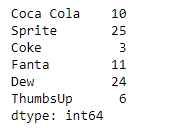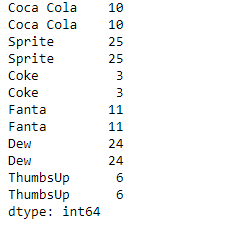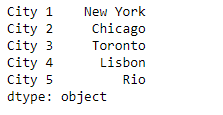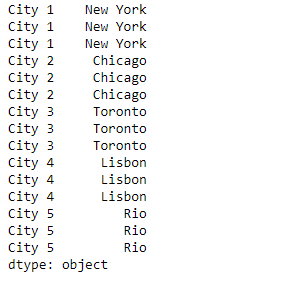# Python | Pandas Series.repeat()

Pandas series is a One-dimensional ndarray with axis labels. The labels need not be unique but must be a hashable type. The object supports both integer- and label-based indexing and provides a host of methods for performing operations involving the index.

Pandas` Series.repeat()` function repeat elements of a Series. It returns a new Series where each element of the current Series is repeated consecutively a given number of times.

Syntax: Series.repeat(repeats, axis=None)

Parameter :
repeats : The number of repetitions for each element.
axis : None

Returns : repeated_series

Example #1: Use `Series.repeat()` function to repeat each value in the given Series object 2 times.

 `# importing pandas as pd ` `import` `pandas as pd ` ` `  `# Creating the Series ` `sr ``=` `pd.Series([``10``, ``25``, ``3``, ``11``, ``24``, ``6``]) ` ` `  `# Create the Index ` `index_ ``=` `[``'Coca Cola'``, ``'Sprite'``, ``'Coke'``, ``'Fanta'``, ``'Dew'``, ``'ThumbsUp'``] ` ` `  `# set the index ` `sr.index ``=` `index_ ` ` `  `# Print the series ` `print``(sr) `

Output :Now we will use `Series.repeat()` function to repeat each value of the given series object 2 times.

 `# repeat twice ` `result ``=` `sr.repeat(repeats ``=` `2``) ` ` `  `# Print the result ` `print``(result) `

Output :As we can see in the output, the `Series.repeat()` function has returned a new series object where each values are repeated the specified number of times.

Example #2 : Use `Series.repeat()` function to repeat each value in the given Series object 3 times.

 `# importing pandas as pd ` `import` `pandas as pd ` ` `  `# Creating the Series ` `sr ``=` `pd.Series([``'New York'``, ``'Chicago'``, ``'Toronto'``, ``'Lisbon'``, ``'Rio'``]) ` ` `  `# Create the Index ` `index_ ``=` `[``'City 1'``, ``'City 2'``, ``'City 3'``, ``'City 4'``, ``'City 5'``]  ` ` `  `# set the index ` `sr.index ``=` `index_ ` ` `  `# Print the series ` `print``(sr) `

Output :Now we will use `Series.repeat()` function to repeat each value of the given series object 3 times.

 `# repeat twice ` `result ``=` `sr.repeat(repeats ``=` `3``) ` ` `  `# Print the result ` `print``(result) `

Output :As we can see in the output, the `Series.repeat()` function has returned a new series object where each values are repeated the specified number of times.

My Personal Notes arrow_drop_upCheck out this Author's contributed articles.

If you like GeeksforGeeks and would like to contribute, you can also write an article using contribute.geeksforgeeks.org or mail your article to contribute@geeksforgeeks.org. See your article appearing on the GeeksforGeeks main page and help other Geeks.

Please Improve this article if you find anything incorrect by clicking on the "Improve Article" button below.

Article Tags :

Be the First to upvote.

Please write to us at contribute@geeksforgeeks.org to report any issue with the above content.